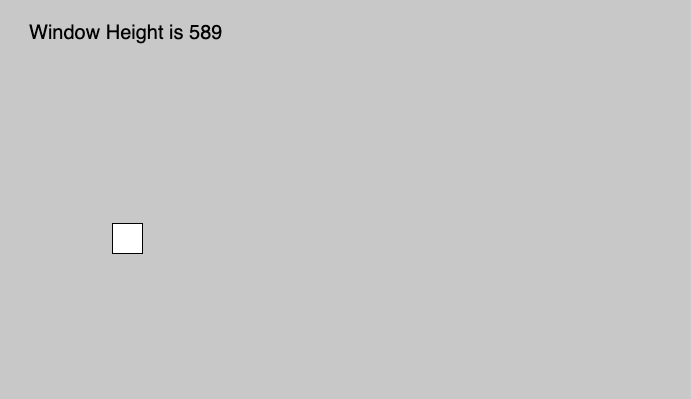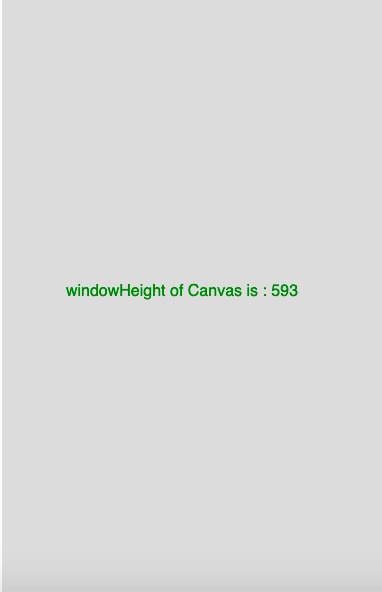GFG App
Open AppBrowser
Continue

The windowHeight in p5.js is a system variable that is used to store the height of the inner window and it maps to window.innerHeight.
Syntax

```windowHeight
```

Parameters: This function does not accept any parameter.

Below program illustrates the windowHeight variable in p5.js:
Example-1:

 `function` `setup() { ` ` `  `    ``createCanvas(1000, 400); ` ` `  `    ``// Set text size to 40px ` `    ``textSize(20); ` `} ` ` `  `function` `draw() { ` `    ``background(200); ` `    ``rect(mouseX, mouseY, 30, 30); ` ` `  `    ``//Use of windowHeight Variable ` `    ``text(``"Window Height is "` `+ windowHeight, 30, 40); ` `} `

Output:Example-2:

 `function` `setup() { ` ` `  `    ``// set height to window height  ` `    ``height = windowHeight; ` ` `  `    ``//create Canvas of size 380*80  ` `    ``createCanvas(380, height); ` `} ` ` `  `function` `draw() { ` `    ``background(220); ` `    ``textSize(16); ` `    ``textAlign(CENTER); ` `    ``fill(color(``'Green'``)); ` ` `  `    ``//use of windowHeight variable ` `    ``text(``"windowHeight of Canvas is : "` `         ``+ height, 180, height / 2); ` `} `

Output:My Personal Notes arrow_drop_up
Related Tutorials